# CBSE Important Questions for Class 12 Physics Chapter 1## myCBSEguide App

Complete Guide for CBSE Students

NCERT Solutions, NCERT Exemplars, Revison Notes, Free Videos, CBSE Papers, MCQ Tests & more.

CBSE Important Questions for Class 12 Physics Chapter 1. We know Physics is tough subject within the consortium of science subjects physics is an important subject. But if you want to make career in these fields like IT Consultant, Lab Technician, Laser Engineer, Optical Engineer etc. You need to have strong fundamentals in physics to crack the exam. myCBSEguide has just released Chapter Wise Question Answers for class 12 Physics. There chapter wise Extra Questions with complete solutions are available for download in myCBSEguide website and mobile app. These Questions with solution are prepared by our team of expert teachers who are teaching grade in CBSE schools for years. There are around 4-5 set of solved Physics Extra Questions from each and every chapter. The students will not miss any concept in these Chapter wise question that are specially designed to tackle Board Exam. We have taken care of every single concept given in CBSE Class 12 Physics syllabus and questions are framed as per the latest marking scheme and blue print issued by CBSE for class 12.

Class 12 Physics Extra Questions

## Electric Charges and Fields Class 12 Physics Extra Questions

Class – 12 Physics (Electric Charges and Fields)

1. For a thin spherical shell of uniform surface charge density {tex}\sigma {/tex} , The magnitude of {tex}\vec{E}{/tex} at a distance r, when r > R (radius of shell) is

1. {tex}E =\frac{4\pi R^2 \sigma}{4\pi \epsilon_0 r^3}{/tex}
2. {tex}E =\frac{4\pi R^2 \sigma }{4\pi \epsilon_0 r^2}{/tex}
3. {tex} E =\frac{R^2 \sigma }{4\pi \epsilon_0 r^2}{/tex}
4. {tex}E =\frac{4\pi R \sigma ^2}{4\pi \epsilon_0 r^2}{/tex}
2. Two insulated charged copper spheres A and B have their centres separated by a distance of 50 cm. What is the mutual force of electrostatic repulsion if the charge on each is {tex}{\rm{6}}.{\rm{5 }} \times {\rm{ 1}}{0^{-{\rm{7}}}}{\rm{C}}{/tex}? The radii of A and B are negligible compared to the distance of separation.

1. {tex}{\rm{3}}.{\rm{5 }} \times {\rm{ 1}}{0^{-{\rm{2}}}}{\rm{N}}{/tex}
2. {tex}{\rm{4}}.{\rm{5 }} \times {\rm{ 1}}{0^{-{\rm{2}}}}{\rm{N}}{/tex}
3. {tex}{\rm{1}}.{\rm{5 }} \times {\rm{ 1}}{0^{-{\rm{2}}}}{\rm{N}}{/tex}
4. {tex}{\rm{2}}.{\rm{5 }} \times {\rm{ 1}}{0^{-{\rm{2}}}}{\rm{N}}{/tex}
3. A conducting sphere of radius 5 cm is charged to 15 {tex}\mu {/tex}C. Another uncharged sphere of radius 10 cm is allowed to touch it for enough time. After the two are separated, the surface density of charge on the two spheres will be in the ratio

1. 2:1
2. 1:2
3. 1:1
4. 3:1
4. The unit of charge is

1. volt
2. ohm
3. coulomb
4. ampere
5. An electric dipole is

1. a pair of electric charges of equal magnitude q but positive sign, separated by a distance d
2. a pair of electric charges of equal magnitude q but opposite sign, separated by a distance
3. a pair of electric charges of equal magnitude q but negative sign, separated by a distance d
4. a pair of electric charges of equal magnitude q separated by a distance d
6. Which orientation of an electric dipole in a uniform electric field would correspond to stable equilibrium?

7. Is the mass of a body affected on charging?

8. Two point charges of {tex}3\mu C{/tex} each are 100 cm apart. At what point on the line joining the charges will the electric intensity be zero?

9. What is the basic cause of quantisation of charge?

10. Calculate the Coulomb force between {tex}2\alpha {/tex} particles separated by {tex}3.2 \times {10^{ – 15}}m{/tex}.

11. An electric dipole is placed in a uniform electric field E with its dipole moment p parallel to the field. then find

1. The work done in turning the dipole till its dipole moment points in the direction opposite to E.
2. The orientation of the dipole for which the torque acting on it becomes maximum.
12. Define the term electric dipole moment. Is it a scalar or vector? Deduce an expression for the electric field at a point on the equatorial plane of an electric dipole of length 2a.

13. Define the term electric field intensity. Write its SI unit. Derive an expression for the electric field intensity at a point on the axis of an electric dipole.

14. Two infinitely large plane thin parallel sheets having surface charge densities {tex}\sigma _ { 1 }{/tex} and {tex}\sigma _ { 2 } \left( \sigma _ { 1 } > \sigma _ { 2 } \right){/tex} are shown in the figure. Write the magnitudes and directions of the net fields in the regions marked II and III.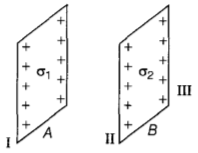15. A thin insulating rod of length L carries a uniformly distributed charge Q. Find the electric field strength at a point along its axis at a distance ‘a’ from one end.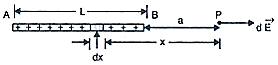Class – 12 Physics (Electric Charges and Fields​​​​​)

1. {tex}E = \frac { 4 \pi R ^ { 2 } \ddot { I } } { 4 \pi \epsilon _ { 0 } r ^ { 2 } }{/tex}
Explanation: Electric field due to hollow sphere or thin spherical shell: Let a spherical conductor of radius R and a charge q is distributed uniformly over it (a) At external point—The charge at the surface of radius r is q the effect of their charge is also spherical, let the Gaussian surface is of radius r, let a small element on the surface whose area vector is dS and electric field is E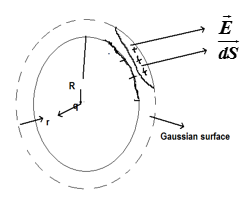{tex}\phi = E\oint dS{/tex} (area of sphere of radius r) {tex}= E\left( {4\pi {r^2}} \right){/tex}
But by Gauss theorem {tex}\phi = \frac{q}{{{\varepsilon _0}}}{/tex}
So {tex}\frac{q}{{{\varepsilon _0}}}{/tex} = E{tex}\times{/tex}{tex}4\pi {r^2}{/tex}
so E = {tex}\frac{q}{{4\pi {\varepsilon _0}{r^2}}}{/tex}
But {tex}\sigma = \frac{q}{{4\pi {R^2}}}{/tex}
so {tex}q = \sigma .4\pi {R^2}{/tex}
So E = {tex}\frac{{q\sigma .4\pi {R^2}}}{{4\pi {\varepsilon _0}{r^2}}} = \frac{{\sigma .4\pi {R^2}}}{{4\pi {\varepsilon _0}{r^2}}}{/tex}
1. {tex}1.5 \times 10 ^ { – 2 } \mathrm { N }{/tex}
Explanation: {tex}F = \frac{1}{{4\pi {\varepsilon _0}}}\frac{{\left| {{q_1}} \right|\left| {{q_2}} \right|}}{{{r^2}}}{/tex} {tex}= \frac{{9 \times {{10}^9} \times {{\left( {6.5 \times {{10}^{ – 7}}} \right)}^2}}}{{{{\left( {50 \times {{10}^{ – 2}}} \right)}^2}}}{/tex}
{tex} = 1.5 \times {10^{ – 2}}N{/tex}
1. 2:1
Explanation: {tex}{q_1} = 4\pi {\varepsilon _0}{r_1}V,\;{q_2} = 4\pi {\varepsilon _0}{r_2}V(by\,q = CV){/tex}
{tex}{\sigma _1} = \frac{{{q_1}}}{{4\pi r_1^2}};\;{\sigma _2} = \frac{{{q_2}}}{{4\pi r_2^2}}{/tex}
{tex}\frac{{{\sigma _1}}}{{{\sigma _2}}} = \left( {\frac{{{q_1}}}{{{q_2}}}} \right)\left( {\frac{{r_2^2}}{{r_1^2}}} \right){/tex}
{tex} = \frac{{{r_2}}}{{{r_1}}} = \frac{{10}}{5} = 2:1{/tex}
1. coulomb
Explanation: The SI unit of quantity of electric charge is the coulomb, which is equivalent to about 6.242×1018 e (e is the charge of a proton).
Hence, the charge of an electron is approximately −1.602×10−19 C. The coulomb is defined as the quantity of charge that has passed through the cross section of an electrical conductor carrying one ampere within one second. The symbol Q is often used to denote a quantity of electricity or charge.
1. a pair of electric charges of equal magnitude q but opposite sign, separated by a distance
Explanation: An electric dipole is a separation of positive and negative charges. The simplest example of this is a pair of electric charges of equal magnitude but opposite sign, separated by some (usually small) distance. A permanent electric dipole is called an electret.
1. The dipole is in stable equilibrium when direction of electric dipole moment of electric dipole is in the direction of electric field.
2. Yes, very slightly. The negatively charged body gains mass also along with electrons.
3. The electric intensity will be zero at a point mid-way between the two charges.
4. Transfer of only integral number of electrons from one body to the other is the basic cause of quantisation of charge.
5. {tex}F = \frac{{9 \times {{10}^9} \times 2 \times 1.6 \times {{10}^{ – 19}} \times 2 \times 1.6 \times {{10}^{ – 19}}}}{{3.2 \times {{10}^{ – 15}} \times 3.2 \times {{10}^{ – 15}}}}N = 90N{/tex}
6. If a dipole is placed in a Electric field then in order to rotate it we have to do the work against Electric field lines which can be find as -1. Work done in rotating the dipole, {tex}W = \int _ { \theta _ { 1 } } ^ { \theta _ { 2 } } \tau d \theta{/tex}
If the dipole is turned from direction parallel to electric field to direction opposite to electric field, then angle {tex}\theta {/tex} will change from 0 to {tex}\pi {/tex}.2. We know that, {tex}\tau = p E \sin \theta{/tex}
If {tex}\theta = \pi / 2{/tex} , then {tex}\tau {/tex} is maximum
i.e. {tex}\tau = p E \sin \frac { \pi } { 2 } \Rightarrow \tau = p E{/tex} (maximum)
Maximum Torque will be experienced by the dipole when its dipole moment is perpendicular to electric field lines.
7. In practice, a dipole is usually a compound that has two equal and opposite charges at a distance. The size is so small that it is considered a point object. So, we see statements like ‘distance from a dipole’. To avoid any confusion, it is convened that ‘distance from a dipole’ would mean ‘distance from the center of the dipole’.Electric dipole moment of an electric dipole is equal to the product of its either charge and the length of the electric dipole.
It is denoted by p.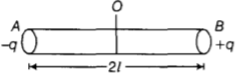p = q {tex} \times {/tex} 2 l
here q is the charge and 2l is the distance between the charges here unit of charge is coulomb and of distance is metre so unit of dipole moment is C-m
It is a vector quantity and its direction is from a negative charge to positive charge(-q to +q).
Let an electric dipole AB consists of two charges +q and -q separated by a distance 2a. We have to find electric field at point P on an equitorial plane separated by a distance r.
Electric field at point P due to charge +q.
{tex}E _ { 1 } = \frac { 1 } { 4 \pi \varepsilon _ { 0 } } \times \frac { q } { \left[ \sqrt { r ^ { 2 } + a ^ { 2 } } \right) ] ^ { 2 } }{/tex}
{tex}E1= \frac { 1 } { 4 \pi \varepsilon _ { 0 } } \times \frac { q } { \left( r ^ { 2 } + a ^ { 2 } \right) }{/tex}
Along with AP as shown in the diagram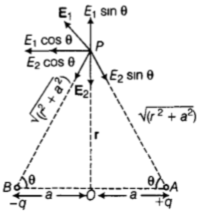Electric field at point P due to charge -q placed at B
{tex}E _ { 2 } = \frac { 1 } { 4 \pi \varepsilon _ { 0 } } \times \frac { q } { r ^ { 2 } + a ^ { 2 } }{/tex} along PB
On resolving E1 and E2 into rectangular components, we get the resultant electric field at point P.
here vertical components of electric field will cancel each other so resultant electric field will be towards left which can be calculated as –
{tex}E = E _ { 1 } \cos \theta + E _ { 2 } \cos \theta{/tex}
{tex}= \frac { 1 } { 4 \pi \varepsilon _ { 0 } } \times \frac { q } { \left( r ^ { 2 } + a ^ { 2 } \right) } \cos \theta + \frac { 1 } { 4 \pi \varepsilon _ { 0 } } \times \frac { q } { \left( r ^ { 2 } + a ^ { 2 } \right) } \cos \theta{/tex}
{tex}= 2 \times \frac { 1 } { 4 \pi \varepsilon _ { 0 } } \times \frac { q } { \left( r ^ { 2 } + a ^ { 2 } \right) } \times \frac { a } { \sqrt { \left( r ^ { 2 } + a ^ { 2 } \right) } }{/tex}
{tex}= \frac { 1 } { 4 \pi \varepsilon _ { 0 } } \times \frac { q 2 a } { \left( r ^ { 2 } + a ^ { 2 } \right) ^ { 3 / 2 } } \quad \text { [But } q \times 2 a = p ]{/tex}
{tex}\therefore E = \frac { 1 } { 4 \pi \varepsilon _ { 0 } } \times \frac { p } { \left( r ^ { 2 } + a ^ { 2 } \right) ^ { 3 / 2 } }{/tex}
If r >> a,
then {tex}E = \frac { 1 } { 4 \pi \varepsilon _ { 0 } } \times \frac { p } { r ^ { 3 } }{/tex}
This equation shows that Electric field due to dipole at a distance “r” from its perpendicular bisector decreases with r and proportional to dipole moment.
8. The force experienced by a unit positive charge placed at a point is termed as the electric field intensity at that point. It is vector quantity it’s direction is in the force acting on +ve charge.
The SI unit of electric field intensity is NC-1
Electric field at an axial point of electric dipole:
Assume point P is located at distance r from the centre of an electric dipole as shown in the figure.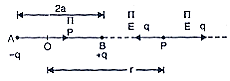Electric field at point P is {tex}\overrightarrow E = {\overrightarrow E _{ + q}} + {\overrightarrow E _{ – q}}{/tex}
{tex}\overrightarrow E = \frac{1}{{4\pi {\varepsilon _0}}}\left[ {\frac{q}{{{{(r – a)}^2}}} – \frac{q}{{{{(r + a)}^2}}}} \right]\widehat p{/tex}
{tex}\overrightarrow E = \frac{q}{{4\pi {\varepsilon _0}}}\left[ {\frac{{{{(r + a)}^2} – {{(r – a)}^2}}}{{({r^2} – {a^2})}}} \right]\widehat p{/tex}
or {tex}\overrightarrow E = \frac{q}{{4\pi {\varepsilon _0}}}\left[ {\frac{{4ra}}{{{{({r^2} – {a^2})}^2}}}} \right]\widehat p{/tex}
or {tex}\overrightarrow E = \frac{1}{{4\pi {\varepsilon _0}}}\frac{{2pr}}{{{{({r^2} – {a^2})}^2}}}\widehat p{/tex} [ p = q (2a)] or {tex}\overrightarrow E = \frac{1}{{4\pi {\varepsilon _0}}}\frac{{2p}}{{{r^3}}}\widehat p{/tex}
or {tex}\overrightarrow E = \frac{1}{{4\pi {\varepsilon _0}}}\frac{{2p}}{{{r^3}}}{/tex} [ For r > > a ]
9. According to the figure, A and B are two thin plane parallel sheets of charge having uniform densities {tex}\sigma _ { 1 }{/tex} and {tex}\sigma _ { 2 }{/tex} with {tex}\sigma _ { 1 } > \sigma _ { 2 }{/tex}
{tex}\phi = E \times{/tex} area of the end faces of the cylinder
{tex}E \times 2 A = \frac { \sigma A } { \varepsilon _ { 0 } } \Rightarrow E = \frac { \sigma } { 2 \varepsilon _ { 0 } }{/tex}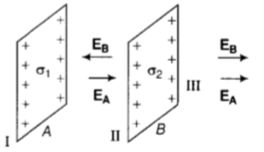In region II –
The electric field due to the sheet of charge A having surface charge density’σ1‘ Will be from left to right (along the positive direction) and that due to the sheet of charge B having surface charge density ‘σ2‘ will be from right to left (along the negative direction) Therefore, in region II, we have electric field opposing each other.
{tex}E = \frac { \sigma _ { 1 } } { 2 \varepsilon _ { 0 } } + \left( – \frac { \sigma _ { 2 } } { 2 \varepsilon _ { 0 } } \right){/tex}
{tex}\Rightarrow \quad E = \frac { 1 } { 2 \varepsilon _ { 0 } } \left( \sigma _ { 1 } – \sigma _ { 2 } \right){/tex} (along positive direction i.e towards right side)
In region III –
The electric fields due to both the charged sheets will be from left to right, i.e. along the positive direction. Therefore, in region III, we have electric fields supporting each other
{tex}E = \frac { \sigma _ { 1 } } { 2 \varepsilon _ { 0 } } + \frac { \sigma _ { 2 } } { 2 \varepsilon _ { 0 } }{/tex}
{tex}\mathbf { E } = \frac { 1 } { \varepsilon _ { 0 } } \left( \sigma _ { 1 } + \sigma _ { 2 } \right){/tex}(along positive direction i.e towards right side)
10. Let us consider an infinitesimal element of length dx at a distance x from the point P. The charge on this element is {tex}dq = \lambda dx{/tex}, where {tex}\lambda = \left( {\frac{Q}{L}} \right){/tex} is the linear charge density.
The magnitude of the electric field at P due to this element is:
{tex}dE = \frac{1}{{4\pi {\varepsilon _0}}}\frac{{dq}}{{{x^2}}} = \frac{1}{{4\pi {\varepsilon _0}}}\frac{{(\lambda dx)}}{{{x^2}}}{/tex}
and its direction is to the right since {tex}\lambda{/tex} is positive. The total electric field strength E is given by:
{tex}E = \frac{1}{{4\pi {\varepsilon _0}}}\lambda \int\limits_a^{a + L} {\frac{{dx}}{{{x^2}}}}{/tex}
{tex} = \frac{\lambda }{{4\pi {\varepsilon _0}}}\left[ { – \frac{1}{x}} \right]_a^{a + L}{/tex}
{tex} = \frac{\lambda }{{4\pi {\varepsilon _0}}}\left[ {\frac{1}{a} – \frac{1}{{a + L}}} \right]{/tex}
{tex} = \frac{Q}{{(4\pi {\varepsilon _0})a(a + L)}}{/tex} {tex}\left( {\because Q = \lambda L} \right){/tex}

## Chapter Wise Extra Questions of Class 12 Physics Part I & Part II## Test Generator

Create Papers with your Name & Logo